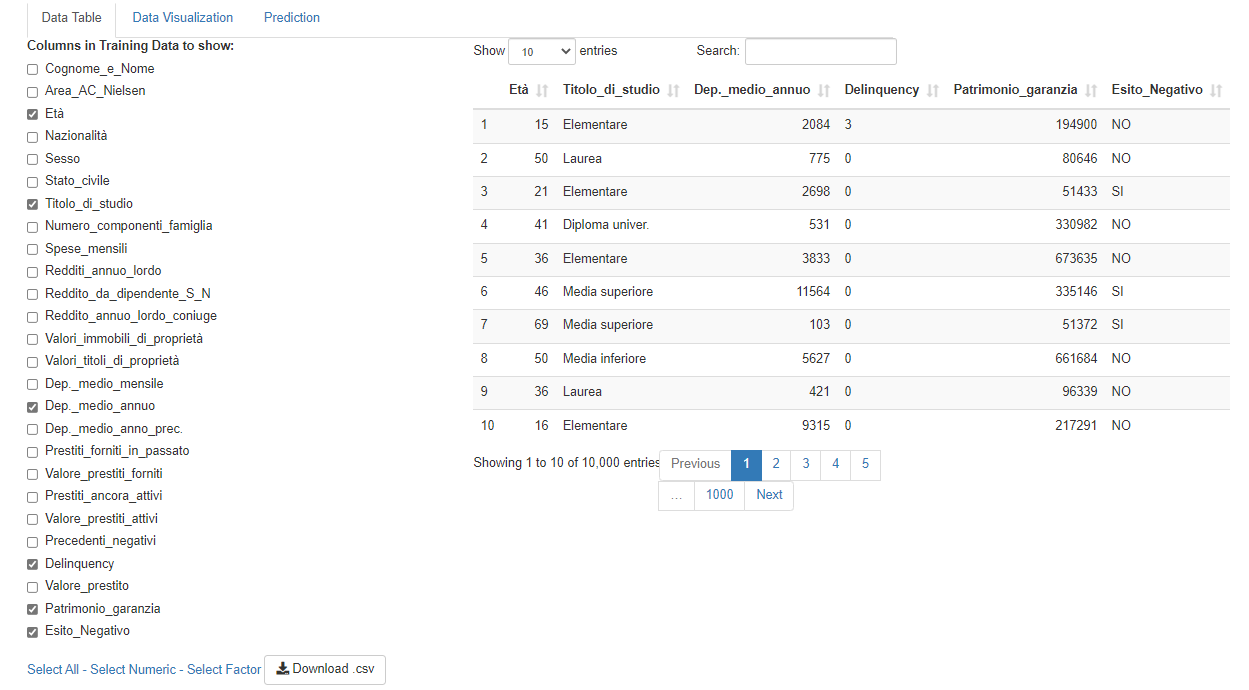﻿

## The Case Study and the R-Shiny App

The Case Study is based on a hypothetical bank credit risk data set.
The data set contains 26 variables collected on 10,000 observations:

1. 13 numerical
2. 13 categorical, including the target variable “Esito Negativo”.

The goal of the Case Study is to analyze the data and to predict the Target Variable.

Click here to see the list of the variables of this Case Study, their possible codes and their English Translation.

The present study is developed in R language. R is an open source programming language specific for statistical data analysis. In particular, the Case Study application is developed with an R package, “Shiny”, that allows to build interactive web apps using R code. The aim of the application is to visualize interactively the data and the results of the analysis of the data set focused on the study of bank credit risk.

Among the models tested to predict the default indicators there are the following:

1. glm = Generalized Linear Model (Logistic Regression)
2. glm_s = Generalized Linear Model (Logistic Regression) with Stepwise Selection
3. random forest = Random Forest
4. bagging = Bagged CART (Classification And Regression Tree)
5. gbm = Stochastic Gradient Boosting Model
6. svm = Support Vector Machine with Linear Kernel
7. ksvm = Support Vector Machine with Radial Kernel
8. nnet = Neural Network, Single Layer
9. mlp = Neural Network, Multi-Layer Perceptron
10. mlpw = Neural Network, Multi-Layer Perceptron with weight Decay
11. pcannet = Neural Network with Principal Components Analysis
12. glmnet = with Glmnet package

The developed R Shiny app is currently available at this web link: https://dev1ratio.shinyapps.io/shiny01/

The app is composed by three pages/tabs.

## First tab: Data Table

The first page allows the visualization of the values of each column of the data set.

It is possible to choose the variables of interest, the number of observations displayed on the screen (10,25,50,100) and sort the columns into multiple levels. It is also possible to download the data in .csv format.Click on each picture to enlarge it.

## Second tab: Data Visualization

The second page allows you to consult the graphs and correlations (or associations) both jointly between the explanatory variables, and as a function of the "Esito Negativo" response variable, that is always present in each graph.

It is possible to choose two by two the variables to be shown on the screen, and graphs with marginal distributions and an appropriate joint distribution plot will be returned depending on the type of variables chosen (categorical, numeric or a categorical and a numeric), always coloured according to the response variable.

Optionally, you can remove zero and missing values.

Click on each picture to enlarge it.

## Third tab: Prediction

The third page shows the performance measures of some models used in the training and forecasting phase.

Box and Whisker plots (bwplots) make a graphical comparison between accuracy, specificity and sensitivity calculated using a repeated 5-fold-cross-validation and dotplots that show the confidence intervals of the average of the calculated indicators.

Finally, it is reported the confusion matrix related just to the most performing model, the GBM (Stochastic Gradient Boosting Model).

Click on each picture to enlarge it.